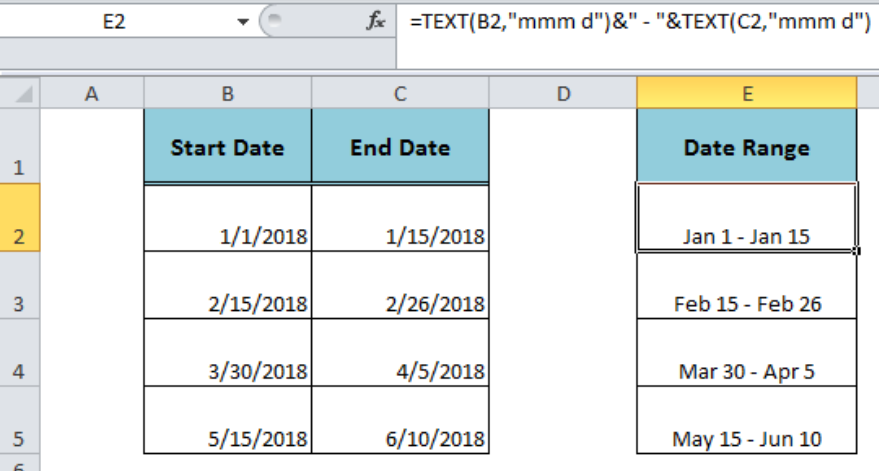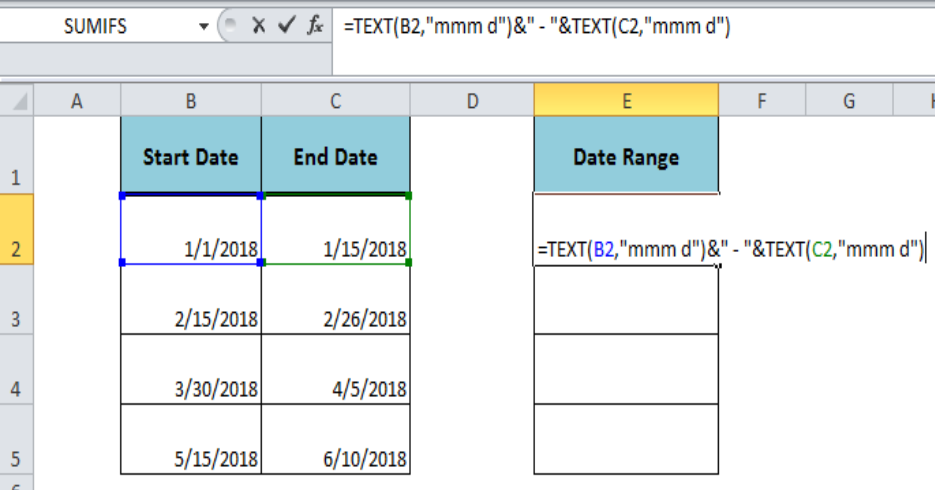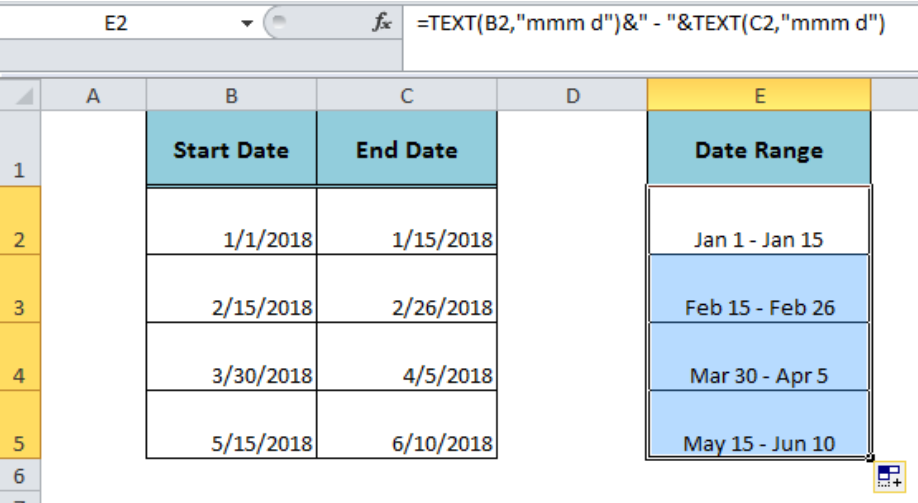Get instant live expert help with Excel or Google Sheets“My Excelchat expert helped me in less than 20 minutes, saving me what would have been 5 hours of work!”

#### Post your problem and you’ll get expert help in seconds.

Your message must be at least 40 characters
Our professional experts are available now. Your privacy is guaranteed.

# Create date range from two dates

When we have two dates in two different cell references and wish to display them in one cell as date range as per our desired format then we will learn how to do that in this article. Using a formula based on the TEXT function and Ampersand (&), concatenating operator, we can create date range from two dates in Excel. This article will step through the process.Figure 1. Creating Date Range From Two Dates

## Formula Syntax

The generic syntax for the formula is as follows;

`=TEXT(date1,"format")&" - "&TEXT(date2,"format")`

Suppose we have the start date in cell B2 and the end date in cell C2. We want to display these both dates in a cell E2 as date range as per a custom date format “mmm d”. Following the above formula syntax, we can create date range from two dates in a single formula, such as;

`=TEXT(B2,"mmm d")&" - "&TEXT(C2,"mmm d")`Figure 2. Applying the Formula to Create Date Range

## How Formula Works

When we have a date value, the TEXT function returns it as a custom date format. By using the TEXT function we can return these two date values in a custom format and with the help of Ampersand (&), concatenating operator, we can combine these two formats in a single formula. After applying the formula, press Enter and copy the formula down to other cells.Figure 3. Displaying Date Range in Single Cell

## Instant Connection to an Expert through our Excelchat Service

Most of the time, the problem you will need to solve will be more complex than a simple application of a formula or function. If you want to save hours of research and frustration, try our live Excelchat service! Our Excel Experts are available 24/7 to answer any Excel question you may have. We guarantee a connection within 30 seconds and a customized solution within 20 minutes.

### Did this post not answer your question? Get a solution from connecting with the expert.Another blog reader asked this question today on Excelchat:
Solution examplesGood morning, I need a cell formatting solution to display leading zero in my excel table with the following conditions. 1. Cannot format as text 2. cell size is not fixed. Please let me know if there is a solution.Thanks in advance
Solved by V. C. in 11 minslooking for an Iferror formula that will bring back a blank value if a date is not listed. Currently using the following but want to add an Iferror statement: =TEXT(H2,"DDDD"). H2 is the raw date ex. 2/8/18. I tried =IFERROR(TEXT(H2,"DDDD"),"") but it keeps returning "saturday" instead of a blank cell. Any help is appreciated.
Solved by F. Q. in 20 minsHow do I make this formula =Value(IFerror(TEXT(AM3,"HMM"),"0")) Return a value of 2400 if the value is not an error?
Solved by F. J. in 21 minsI have an excel spreadsheet with two worksheets. And the following formula is not pulling the data. =VLOOKUP(\$A\$2:\$A\$566,'Module Type Info'!\$A\$2:\$D\$97,4,FALSE) In sheet 1, I am using all data in column 1 for the lookup In sheet 2, I have selected the first 4 columns of data for array I want to pull data from sheet 2, column 4 into sheet 1 Both tabs are sorted alphabetically. Confirmed that the value in column 4 of sheet 2 is a TEXT field. Why is this not pulling the info from sheet 2 into sheet 1
Solved by F. L. in 39 minsI need to add an formula into this one: =IF(\$L\$1="","",VLOOKUP(TEXT(\$L\$1,"000000"),'FRS102 BASE & GBP TB MAY 2018'!\$E:\$X,MATCH(A25,'FRS102 BASE & GBP TB MAY 2018'!\$Q\$3:\$X\$3,0)+3,0))
Solved by K. D. in 11 mins## Subscribe to Excelchat.coAnother blog reader asked this question today on Excelchat: